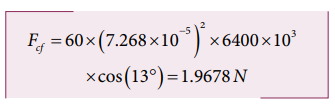Home | | Physics 11th std | Solved Example Problems for Centrifugal Force due to Rotation of the Earth

# Solved Example Problems for Centrifugal Force due to Rotation of the Earth

Physics : Laws of Motion : Centrifugal Force due to Rotation of the Earth

Solved Example Problems for Centrifugal Force due to Rotation of the Earth

### Example 3.26

Calculate the centrifugal force experienced by a man of 60 kg standing at Chennai? (Given: Latitude of Chennai is 13°

### Solution

The  centrifugal force is given by Fc = mω2 R cosθ

The angular velocity (ω) of Earth = 2π/T.

where T is time period of the Earth (24 hours)The radius of the Earth R = 6400 Km = 6400 × 103 m

Latitude of Chennai =13°A 60 kg man experiences centrifugal force of approximately 2 Newton. But due to Earth’s gravity a man of 60 kg experiences a force =mg = 60 × 9.8 = 588N. This force is very much larger than the centrifugal force.

Study Material, Lecturing Notes, Assignment, Reference, Wiki description explanation, brief detail
11th Physics : UNIT 3 : Laws of Motion : Solved Example Problems for Centrifugal Force due to Rotation of the Earth |